# Extendible Hashing (Dynamic approach to DBMS)

• Difficulty Level : Medium
• Last Updated : 22 Mar, 2021

Extendible Hashing is a dynamic hashing method wherein directories, and buckets are used to hash data. It is an aggressively flexible method in which the hash function also experiences dynamic changes.

Main features of Extendible Hashing: The main features in this hashing technique are:

• Directories: The directories store addresses of the buckets in pointers. An id is assigned to each directory which may change each time when Directory Expansion takes place.
• Buckets: The buckets are used to hash the actual data.

Basic Structure of Extendible Hashing: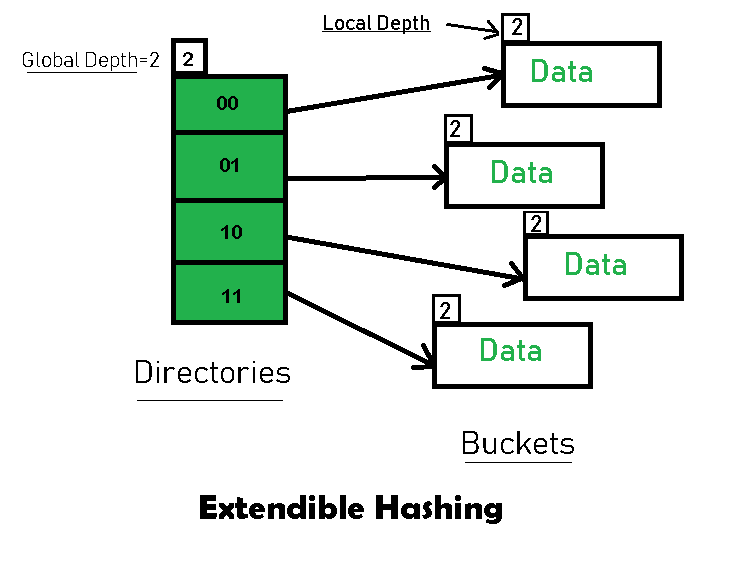Frequently used terms in Extendible Hashing:

• Directories: These containers store pointers to buckets. Each directory is given a unique id which may change each time when expansion takes place. The hash function returns this directory id which is used to navigate to the appropriate bucket. Number of Directories = 2^Global Depth.
• Buckets: They store the hashed keys. Directories point to buckets. A bucket may contain more than one pointers to it if its local depth is less than the global depth.
• Global Depth: It is associated with the Directories. They denote the number of bits which are used by the hash function to categorize the keys. Global Depth = Number of bits in directory id.
• Local Depth: It is the same as that of Global Depth except for the fact that Local Depth is associated with the buckets and not the directories. Local depth in accordance with the global depth is used to decide the action that to be performed in case an overflow occurs. Local Depth is always less than or equal to the Global Depth.
• Bucket Splitting: When the number of elements in a bucket exceeds a particular size, then the bucket is split into two parts.
• Directory Expansion: Directory Expansion Takes place when a bucket overflows. Directory Expansion is performed when the local depth of the overflowing bucket is equal to the global depth.

Basic Working of Extendible Hashing: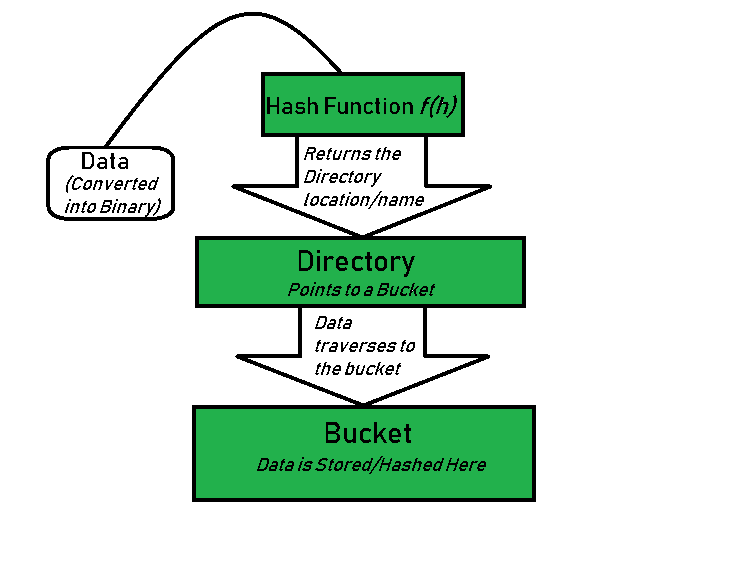• Step 1 – Analyze Data Elements: Data elements may exist in various forms eg. Integer, String, Float, etc.. Currently, let us consider data elements of type integer. eg: 49.
• Step 2 – Convert into binary format: Convert the data element in Binary form. For string elements, consider the ASCII equivalent integer of the starting character and then convert the integer into binary form. Since we have 49 as our data element, its binary form is 110001.
• Step 3 – Check Global Depth of the directory. Suppose the global depth of the Hash-directory is 3.
• Step 4 – Identify the Directory: Consider the ‘Global-Depth’ number of LSBs in the binary number and match it to the directory id.
Eg. The binary obtained is: 110001 and the global-depth is 3. So, the hash function will return 3 LSBs of 110001 viz. 001.
• Step 5 – Navigation: Now, navigate to the bucket pointed by the directory with directory-id 001.
• Step 6 – Insertion and Overflow Check: Insert the element and check if the bucket overflows. If an overflow is encountered, go to step 7 followed by Step 8, otherwise, go to step 9.
• Step 7 – Tackling Over Flow Condition during Data Insertion: Many times, while inserting data in the buckets, it might happen that the Bucket overflows. In such cases, we need to follow an appropriate procedure to avoid mishandling of data.
First, Check if the local depth is less than or equal to the global depth. Then choose one of the cases below.
• Case1: If the local depth of the overflowing Bucket is equal to the global depth, then Directory Expansion, as well as Bucket Split, needs to be performed. Then increment the global depth and the local depth value by 1. And, assign appropriate pointers.
Directory expansion will double the number of directories present in the hash structure.
• Case2: In case the local depth is less than the global depth, then only Bucket Split takes place. Then increment only the local depth value by 1. And, assign appropriate pointers.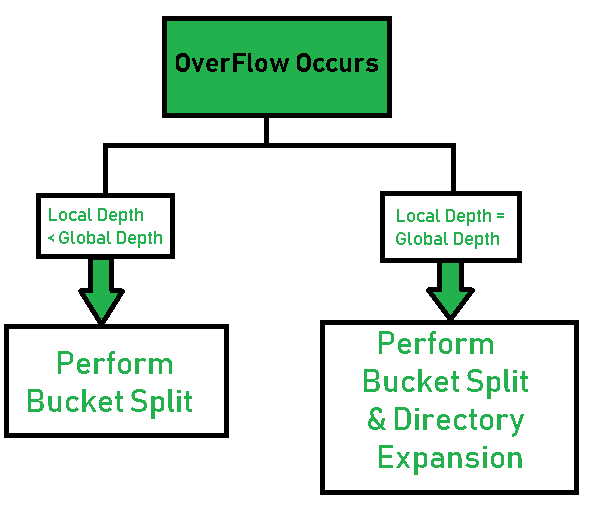• Step 8 – Rehashing of Split Bucket Elements: The Elements present in the overflowing bucket that is split are rehashed w.r.t the new global depth of the directory.
• Step 9 – The element is successfully hashed.

Example based on Extendible Hashing: Now, let us consider a prominent example of hashing the following elements: 16,4,6,22,24,10,31,7,9,20,26.
Bucket Size: 3 (Assume)
Hash Function: Suppose the global depth is X. Then the Hash Function returns X LSBs.

• Solution: First, calculate the binary forms of each of the given numbers.
16- 10000
4- 00100
6- 00110
22- 10110
24- 11000
10- 01010
31- 11111
7- 00111
9- 01001
20- 10100
26- 11010
• Initially, the global-depth and local-depth is always 1. Thus, the hashing frame looks like this: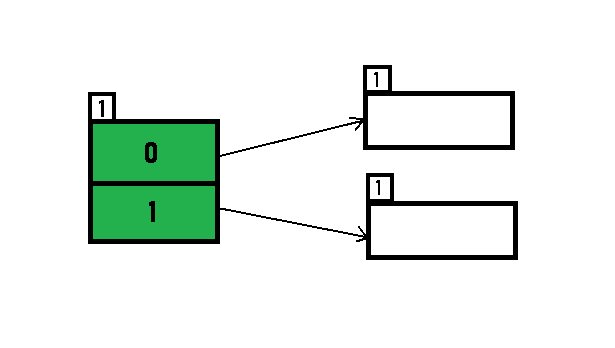• Inserting 16:
The binary format of 16 is 10000 and global-depth is 1. The hash function returns 1 LSB of 10000 which is 0. Hence, 16 is mapped to the directory with id=0.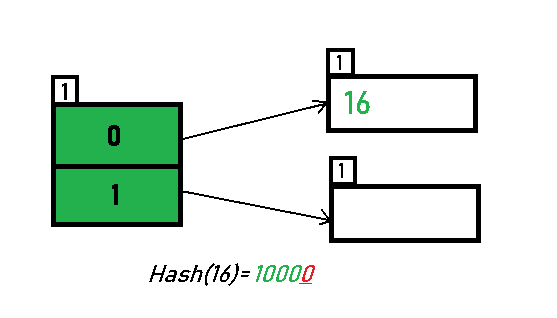• Inserting 4 and 6:
Both 4(100) and 6(110)have 0 in their LSB. Hence, they are hashed as follows: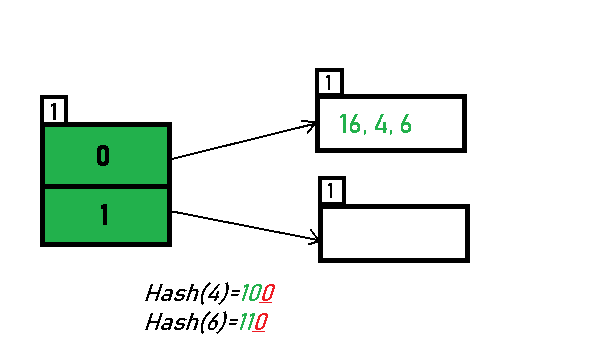• Inserting 22: The binary form of 22 is 10110. Its LSB is 0. The bucket pointed by directory 0 is already full. Hence, Over Flow occurs.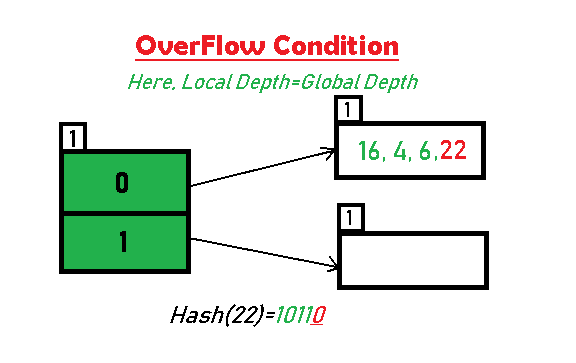• As directed by Step 7-Case 1, Since Local Depth = Global Depth, the bucket splits and directory expansion takes place. Also, rehashing of numbers present in the overflowing bucket takes place after the split. And, since the global depth is incremented by 1, now,the global depth is 2. Hence, 16,4,6,22 are now rehashed w.r.t 2 LSBs.[ 16(10000),4(100),6(110),22(10110) ]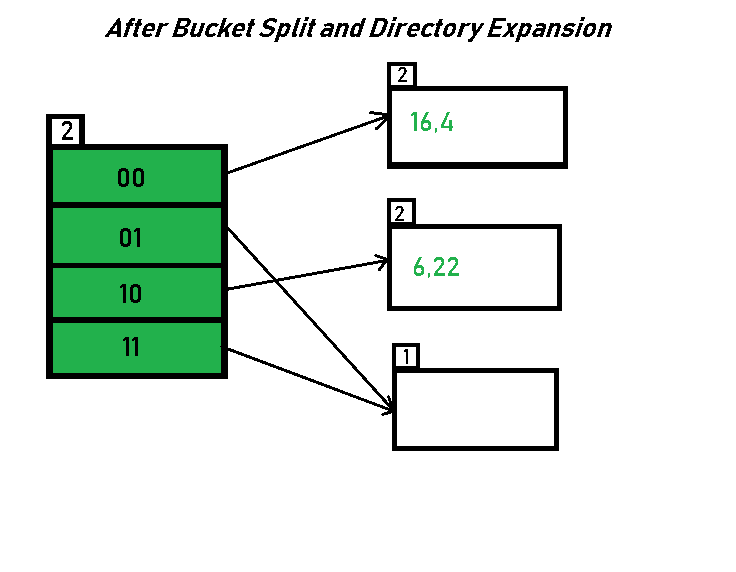•

*Notice that the bucket which was underflow has remained untouched. But, since the number of directories has doubled, we now have 2 directories 01 and 11 pointing to the same bucket. This is because the local-depth of the bucket has remained 1. And, any bucket having a local depth less than the global depth is pointed-to by more than one directories.

• Inserting 24 and 10: 24(11000) and 10 (1010) can be hashed based on directories with id 00 and 10. Here, we encounter no overflow condition.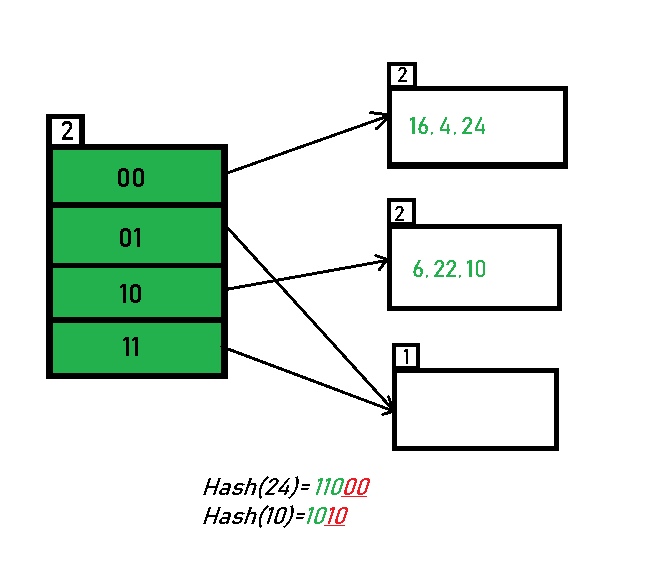• Inserting 31,7,9: All of these elements[ 31(11111), 7(111), 9(1001) ] have either 01 or 11 in their LSBs. Hence, they are mapped on the bucket pointed out by 01 and 11. We do not encounter any overflow condition here.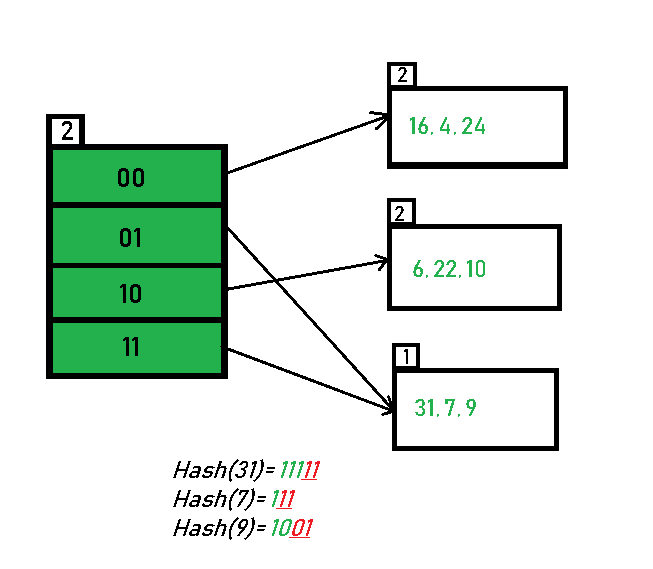• Inserting 20: Insertion of data element 20 (10100) will again cause the overflow problem.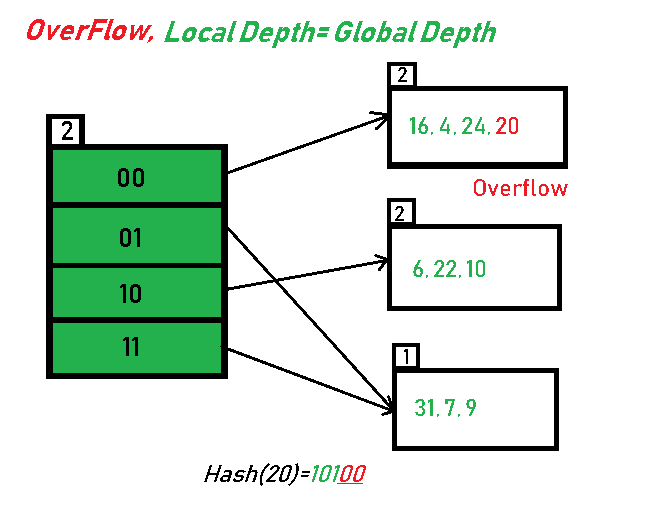• 20 is inserted in bucket pointed out by 00. As directed by Step 7-Case 1, since the local depth of the bucket = global-depth, directory expansion (doubling) takes place along with bucket splitting. Elements present in overflowing bucket are rehashed with the new global depth. Now, the new Hash table looks like this: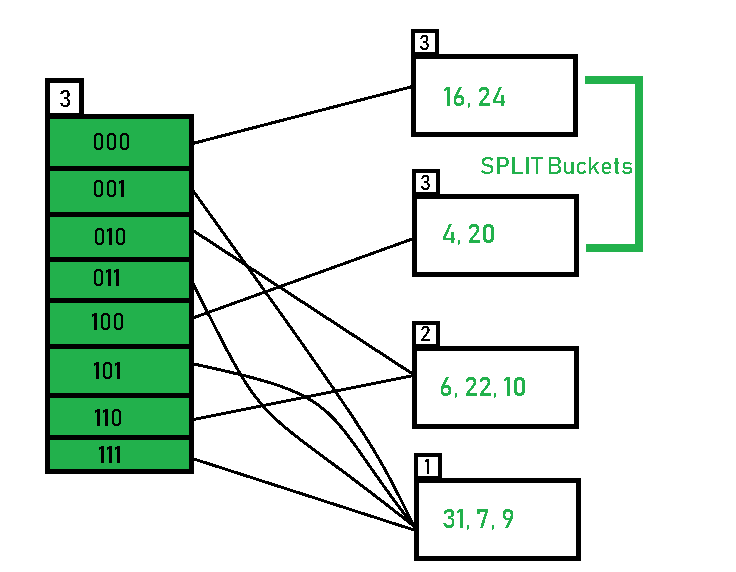• Inserting 26: Global depth is 3. Hence, 3 LSBs of 26(11010) are considered. Therefore 26 best fits in the bucket pointed out by directory 010.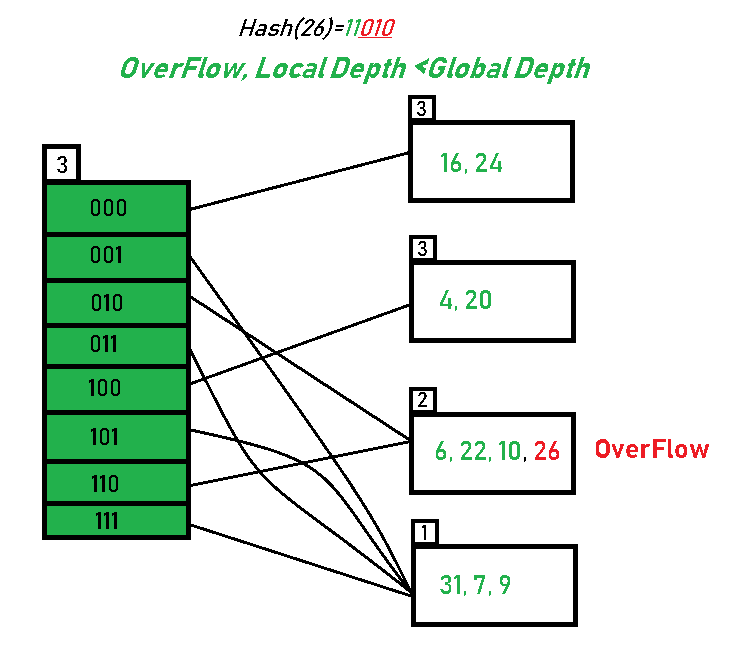• The bucket overflows, and, as directed by Step 7-Case 2, since the local depth of bucket < Global depth (2<3), directories are not doubled but, only the bucket is split and elements are rehashed.
Finally, the output of hashing the given list of numbers is obtained.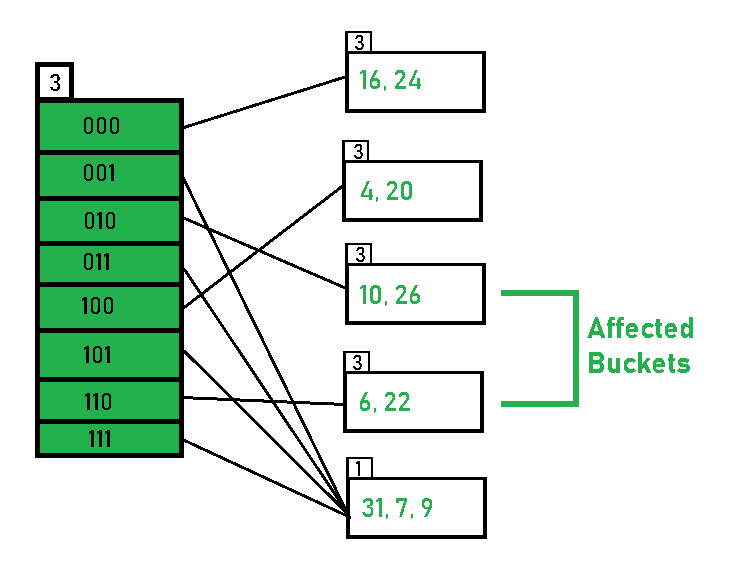• Hashing of 11 Numbers is Thus Completed.

Key Observations:

1. A Bucket will have more than one pointers pointing to it if its local depth is less than the global depth.
2. When overflow condition occurs in a bucket, all the entries in the bucket are rehashed with a new local depth.
3. If Local Depth of the overflowing bucket
4. The size of a bucket cannot be changed after the data insertion process begins.

1. Data retrieval is less expensive (in terms of computing).
2. No problem of Data-loss since the storage capacity increases dynamically.
3. With dynamic changes in hashing function, associated old values are rehashed w.r.t the new hash function.

Limitations Of Extendible Hashing:

1. The directory size may increase significantly if several records are hashed on the same directory while keeping the record distribution non-uniform.
2. Size of every bucket is fixed.
3. Memory is wasted in pointers when the global depth and local depth difference becomes drastic.
4. This method is complicated to code.

Data Structures used for implementation:

My Personal Notes arrow_drop_up